The Australian Journal of Mathematical Analysis and Applications

 Home News Editors Volumes RGMIA Subscriptions Authors Contact

ISSN 1449-5910

You searched for isac
Total of 65 results found in site

19: Paper Source PDF document

Paper's Title:

A relation between nuclear cones and full nuclear cones

Author(s):

G. Isac and A. B. Nemeth

Department of Mathematics,
P. O. Box 17000 STN Forces Kingston, Ontario,
isac-g@rmc.ca

Faculty of Mathematics and Computer Science,
Babes-Bolyai University,
3400 Cluj-Napoca,
Romania.
nemab@math.ubbcluj.ro

Abstract:

The notion of nuclear cone in locally convex spaces corresponds to the notion of well based cone in normed spaces. Using the bipolar theorem from locally convex spaces it is proved that every closed nuclear cone is a full nuclear cone. Thus every closed nuclear cone can be associated to a mapping from a family of continuous seminorms in the space to the topological dual of the space. The relation with Pareto efficiency is discussed.

8: Paper Source PDF document

Paper's Title:

Applications of Relations and Relators in the Extensions of Stability Theorems for Homogeneous and Additive Functions

Author(s):

Árpád Száz

Institute of Mathematics, University of Debrecen,
H-4010 Debrecen, Pf. 12,
Hungary
szaz@math.klte.hu

Abstract:

By working out an appropriate technique of relations and relators and extending the ideas of the direct methods of Z. Gajda and R. Ger, we prove some generalizations of the stability theorems of D. H. Hyers, T. Aoki, Th. M. Rassias and P. Găvruţă in terms of the existence and unicity of 2-homogeneous and additive approximate selections of generalized subadditive relations of semigroups to vector relator spaces. Thus, we obtain generalizations not only of the selection theorems of Z. Gajda and R. Ger, but also those of the present author.

5: Paper Source PDF document

Paper's Title:

Viability Theory And Differential Lanchester Type Models For Combat.
Differential Systems.

Author(s):

G. Isac and A. Gosselin

Department Of Mathematics, Royal Military College Of Canada,
P.O. Box 17000, S
tn Forces, Kingston, Ontario, Canada K7k 7b4

URL
:

URL
:

Abstract:

In 1914, F.W. Lanchester proposed several mathematical models based on differential equations to describe combat situations . Since then, his work has been extensively modified to represent a variety of competitions including entire wars. Differential Lanchester type models have been studied from many angles by many authors in hundreds of papers and reports. Lanchester type models are used in the planning of optimal strategies, supply and tactics. In this paper, we will show how these models can be studied from a viability theory stand point. We will introduce the notion of winning cone and show that it is a viable cone for these models. In the last part of our paper we will use the viability theory of differential equations to study Lanchester type models from the optimal theory point of view.

4: Paper Source PDF document

Paper's Title:

Pseudomonotonicity and Quasimonotonicity by Translations versus Monotonicity in Hilbert Spaces

Author(s):

George Isac and Dumitru Motreanu

Department of Mathematics, Royal Military College of Canada, P.O. Box 17000 Stn Forces

Département de Mathématiques, Université de Perpignan, 66860 Perpignan, France.

Abstract:

Let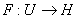be a Gâteaux differentiable mapping on an open convex subset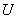of a Hilbert space. If there exists a straight line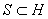such that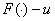is pseudomonotone for any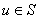then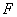is monotone. Related results using a regularity condition are given.

4: Paper Source PDF document

Paper's Title:

A Fixed Point Approach to the Stability of the Equation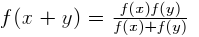Author(s):

Soon-Mo Jung

Mathematics Section, College of Science and Technology
Hong-Ik University, 339-701 Chochiwon
Republic of Korea.

smjung@hongik.ac.kr

Abstract:

We will apply a fixed point method for proving the Hyers--Ulam stability of the functional equation.

3: Paper Source PDF document

Paper's Title:

Equilibria and Periodic Solutions of Projected Dynamical Systems on Sets with Corners

Author(s):

Matthew D. Johnston and Monica-Gabriela Cojocaru

Department of Applied Mathematics, University of Waterloo,
mdjohnst@math.uwaterloo.ca

Department of Mathematics & Statistics, University of Guelph,
mcojocar@uoguelph.ca

Abstract:

Projected dynamical systems theory represents a bridge between the static worlds of variational inequalities and equilibrium problems, and the dynamic world of ordinary differential equations. A projected dynamical system (PDS) is given by the flow of a projected differential equation, an ordinary differential equation whose trajectories are restricted to a constraint set K. Projected differential equations are defined by discontinuous vector fields and so standard differential equations theory cannot apply. The formal study of PDS began in the 90's, although some results existed in the literature since the 70's. In this paper we present a novel result regarding existence of equilibria and periodic cycles of a finite dimensional PDS on constraint sets K, whose points satisfy a corner condition. The novelty is due to proving existence of boundary equilibria without using a variational inequality approach or monotonicity type conditions.

3: Paper Source PDF document

Paper's Title:

On Stan Ulam and his Mathematics

Author(s):

Krzysztof Ciesielski and Themistocles M. Rassias

Mathematics Institute, Jagiellonian University,
Ł
jasiewicza 6, 30-348 Kraków,
Poland
Department of Mathematics. National Technical University of Athens,
Zografou Campus, 15780 Athens,
Greece

Krzysztof.Ciesielski@im.uj.edu.pl
trassias@math.ntua.gr

Abstract:

In this note we give a glimpse of the curriculum vitae of Stan Ulam, his personality and some of the mathematics he was involved in.

3: Paper Source PDF document

Paper's Title:

On the Generalized Stability and Asymptotic Behavior of Quadratic Mappings

Author(s):

Hark-Mahn Kim, Sang-Baek Lee and Eunyoung Son

Department of Mathematics
Chungnam National University
Daejeon, 305-764,
Republic of Korea

hmkim@cnu.ac.kr

Abstract:

We extend the stability of quadratic mappings to the stability of general quadratic mappings with several variables, and then obtain an improved asymptotic property of quadratic mappings on restricted domains.

3: Paper Source PDF document

Paper's Title:

Hyers-Ulam-Rassias Stability of a Generalized Jensen Functional Equation

Author(s):

A. Charifi, B. Bouikhalene, E. Elqorachi and A. Redouani

Department of
Mathematics, Faculty of Sciences,
Ibn Tofail University,
Kenitra, Morocco
charifi2000@yahoo.fr bbouikhalene@yahoo.fr

Department of
Mathematics, Faculty of Sciences,
Ibn Zohr University,
elqorachi@hotmail.com Redouani-ahmed@yahoo.fr

Abstract:

In this paper we obtain the Hyers-Ulam-Rassias stability for the generalized Jensen's functional equation in abelian group (G,+). Furthermore we discuss the case where G is amenable and we give a note on the Hyers-Ulam-stability of the K-spherical (n × n)-matrix functional equation.

3: Paper Source PDF document

Paper's Title:

Approximation of an AQCQ-Functional Equation and its Applications

Author(s):

Choonkil Park and Jung Rye Lee

Department of Mathematics,
Research Institute for Natural Sciences,
Hanyang University, Seoul 133-791,
Korea;

Department of Mathematics,
Daejin University,
Kyeonggi 487-711,
Korea

baak@hanyang.ac.kr
jrlee@daejin.ac.kr

Abstract:

This paper is a survey on the generalized Hyers-Ulam stability of an AQCQ-functional equation in several spaces. Its content is divided into the following sections:

1. Introduction and preliminaries.

2. Generalized Hyers-Ulam stability of an AQCQ-functional equation in Banach spaces: direct method.

3. Generalized Hyers-Ulam stability of an AQCQ-functional equation in Banach spaces: fixed point method.

4. Generalized Hyers-Ulam stability of an AQCQ-functional equation in random Banach spaces: direct method.

5. Generalized Hyers-Ulam stability of an AQCQ-functional equation in random Banach spaces: fixed point method.

6. Generalized Hyers-Ulam stability of an AQCQ-functional equation in non-Archi-medean Banach spaces: direct method.

7. Generalized Hyers-Ulam stability of an AQCQ-functional equation in non-Archi-medean Banach spaces: fixed point method.

2: Paper Source PDF document

Paper's Title:

On a Method of Proving the Hyers-Ulam Stability of Functional Equations on Restricted Domains

Author(s):

Janusz Brzdęk

Department of Mathematics
Pedagogical University Podchor
ąźych 2,
30-084 Krak
ów,
Poland
jbrzdek@ap.krakow.pl

Abstract:

We show that generalizations of some (classical) results on the Hyers-Ulam stability of functional equations, in several variables, can be very easily derived from a simple result on stability of a functional equation in single variable

2: Paper Source PDF document

Paper's Title:

Differentiability of Distance Functions in p-Normed Spaces

Author(s):

M. S. Moslehian, A. Niknam, S. Shadkam Torbati

Department of Pure Mathematics, Centre of Excellence in Analysis on Algebraic Structures (CEAAS),,
Iran

moslehian@ferdowsi.um.ac.ir
niknam@math.um.ac.ir

Abstract:

The farthest point mapping in a p-normed space X is studied in virtue of the Gateaux derivative and the Frechet derivative. Let M be a closed bounded subset of X having the uniformly p-Gateaux differentiable norm. Under certain conditions, it is shown that every maximizing sequence is convergent, moreover, if M is a uniquely remotal set then the farthest point mapping is continuous and so M is singleton. In addition, a Hahn--Banach type theorem in \$p\$-normed spaces is proved.

1: Paper Source PDF document

Paper's Title:

Stability of a Pexiderized Equation

Author(s):

Maryam Amyar

Department of Mathematics,
and Banach Mathematical Research Group (BMRG)
amyari@mshdiau.ac.ir

URL: http://amyari.mshdiau.ac.ir

Abstract:

The aim of the paper is to prove the stability of the Pexiderized equation f(x)=g(y+x)-h(y-x), for any amenable abelian group.

1: Paper Source PDF document

Paper's Title:

A Stability of the G-type Functional Equation

Author(s):

Gwang Hui Kim

Department of Mathematics, Kangnam University
Suwon 449-702, Korea.
ghkim@kangnam.ac.kr

Abstract:

We will investigate the stability in the sense of Găvruţă for the G-type functional equation f(φ(x))=Γ(x)f(x)+ψ(x) and the stability in the sense of Ger for the functional equation of the form f(φ(x))=Γ(x)f(x). As a consequence, we obtain a stability results for G-function equation.

1: Paper Source PDF document

Paper's Title:

Ulam Stability of Functional Equations

Author(s):

Stefan Czerwik and Krzysztof Król

Institute of Mathematics
Silesian University of Technology
Kaszubska 23, 44-100 Gliwice,
Poland

Stefan.Czerwik@polsl.pl
Krzysztof.Krol@polsl.pl

Abstract:

In this survey paper we present some of the main results on Ulam-Hyers-Rassias stability for important functional equations.

1: Paper Source PDF document

Paper's Title:

Stability of Almost Multiplicative Functionals

Author(s):

Norio Niwa, Hirokazu Oka, Takeshi Miura and Sin-Ei Takahasi

Faculty of Engineering, Osaka Electro-Communication University,
Neyagawa 572-8530,
Japan

Faculty of Engineering, Ibaraki University,
Hitachi 316-8511,
Japan

Department of Applied Mathematics and Physics, Graduate School of Science and Engineering,
Yamagata University,
Yonezawa 992-8510
Japan

oka@mx.ibaraki.ac.jp
miura@yz.yamagata-u.ac.jp
sin-ei@emperor.yz.yamagata-u.ac.jp

Abstract:

Let δ and p be non-negative real numbers. Let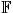be the real or complex number field and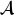a normed algebra over. If a mapping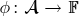satisfies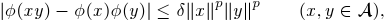then we show that φ is multiplicative or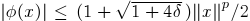for all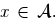If, in addition, φ satisfies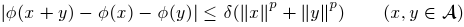for some p1, then by using Hyers-Ulam-Rassias stability of additive Cauchy equation, we show that φ is a ring homomorphism or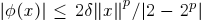for all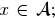In other words, φ is a ring homomorphism, or an approximately zero mapping. The results of this paper are inspired by Th.M. Rassias' stability theorem.

1: Paper Source PDF document

Paper's Title:

Fixed Points and Stability of the Cauchy Functional Equation

Author(s):

Choonkil Park and Themistocles M. Rassias

Department of Mathematics, Hanyang University,
Seoul 133-791,
Republic of Korea

Department of Mathematics, National Technical University of Athens,
Zografou Campus, 15780 Athens,
Greece

baak@hanyang.ac.kr
trassias@math.ntua.gr

Abstract:

Using fixed point methods, we prove the generalized Hyers-Ulam stability of homomorphisms in Banach algebras and of derivations on Banach algebras for the Cauchy functional equation.

1: Paper Source PDF document

Paper's Title:

Ulam Stability of Reciprocal Difference and Adjoint Functional Equations

Author(s):

K. Ravi, J. M. Rassias and B. V. Senthil Kumar

Department of Mathematics,
Sacred Heart College, Tirupattur - 635601,
India

Pedagogical Department E. E.,
Section of Mathematics and Informatics,
National and Capodistrian University of Athens,
Athens, Attikis 15342,
GREECE

Department of Mathematics,
C.Abdul Hakeem College of Engineering and
Technology, Melvisharam - 632 509, India

shckavi@yahoo.co.in
jrassias@primedu.uoa.gr
bvssree@yahoo.co.in

Abstract:

In this paper, the reciprocal difference functional equation (or RDF equation) and the reciprocal adjoint functional equation (or RAF equation) are introduced. Then the pertinent Ulam stability problem for these functional equations is solved, together with the extended Ulam (or Rassias) stability problem and the generalized Ulam (or Ulam-Gavruta-Rassias) stability problem for the same equations.

Search and serve lasted 0 second(s).